## How to Calculate and Solve for Relative Compaction | Soil Mechanics and Foundation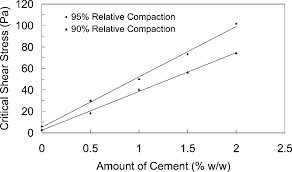The image above represents relative compaction.

To compute for relative compaction, two essential parameters are needed and these parameters are Field dry density (ρd fieldand Maximum dry density (ρd max).

The formula for calculating relative compaction:

Rc = ρd field / ρd max

Where:

Rc = Relative Compaction
ρd field = Field Dry Density
ρd max = Maximum Dry Density

Let’s solve an example;
Find the relative compaction when the field dry density is 44 and the maximum dry density is 11.

This implies that;

ρd field = Field Dry Density = 44
ρd max = Maximum Dry Density = 11

Rc = ρd field / ρd max
Rc = 44 / 11
Rc = 4

Therefore, the relative compaction is 4.

Calculating for the Field Dry Density when the Relative Compaction and the Maximum Dry Density is Given.

ρd field = Rc x ρd max

Where;

ρd field = Field Dry Density
Rc = Relative Compaction
ρd max = Maximum Dry Density

Let’s solve an example;
Find the field dry density when the relative compaction is 25 and the maximum dry density is 4.

This implies that;

Rc = Relative Compaction = 25
ρd max = Maximum Dry Density = 4

ρd field = Rc x ρd max
ρd field = 25 x 4
ρd field = 100

Therefore, the field dry density is 100.
Continue reading How to Calculate and Solve for Relative Compaction | Soil Mechanics and Foundation

## How to calculate for Tractive Force | Soil Mechanics and Foundation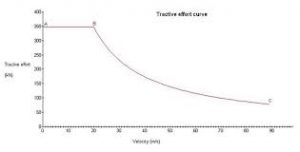The image above represents tractive force.

To compute for tractive force, four essential parameters are needed and these parameters are Unit weight of water (w), Cross-sectional area of the channel (A), Length of the channel (L) and Inclination Angle (θ).

The formula for calculating tractive force:

F = WAL . sinθ

Where:

F = Tractive Force
W = Unit Weight of Water
A = Cross-Sectional Area of the Channel
L = Length of the Channel
θ = Inclination Angle

Let’s solve an example;
Given that the unit weight of water is 4, the cross-sectional area of the channel is 7, the lenght of the channel is 11 and the inclination angel is 9.

This implies that;

W = Unit Weight of Water = 4
A = Cross-Sectional Area of the Channel = 7
L = Length of the Channel = 11
θ = Inclination Angle = 9

F = WAL . sinθ
F = (4)(7)(11) . sin(9°)
F = 308 . 0.156
F = 48.18

Therefore, the tractive force is 48.18.

Calculating for the Cross-Sectional Area of the Channel when the Tractive Force, the Lenght of the Channel, the Unit Weight of Water and the Inclination Angel is Given.

A = F / WL . sin θ

Where;

A = Cross-Sectional Area of the Channel
F = Tractive Force
W = Unit Weight of Water
L = Length of the Channel
θ = Inclination Angle

Let’s solve an example;
Find the cross-sectional area of the channel when the tractive force is 40, the unit weight of water is 2, the length of the channel is 4 and the inclination angle is 6.

This implies that;

F = Tractive Force = 40
W = Unit Weight of Water = 2
L = Length of the Channel = 4
θ = Inclination Angle = 6

A = F / WL . sin θ
A = 40 / (2)(4) . sin 6
A = 40 / (8) . 0.104
A = 40 / 0.832
A = 48.07

Therefore, the cross-sectional area of the channel is 48.07.

## How to Calculate and Solve for Dry Unit Weight of Soil | Soil Mechanics and Foundation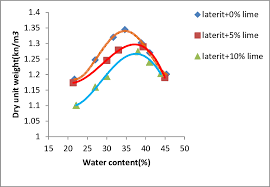The image above represents dry unit weight of soil.

To compute for dry unit weight of soil, two essential parameters are needed and these parameters are Unit weight of soil (γ) and Moisture content (w).

The formula for calculating dry unit weight of soil:

γd = γ / 1 + w

Where:

γd = Dry Unit Weight of Soil
γ = Unit Weight of Soil
w = Moisture Content

Let’s solve an example;
Find the dry unit weight of soil when the unit weight of soil is 12 and the moisture content is 6.

This implies that;

γ = Unit Weight of Soil = 12
w = Moisture Content = 6

γd = γ / 1 + w
γd = 12 / 1 + 6
γd = 12 / 7
γd = 1.71

Therefore, the dry unit weight of soil is 1.71.

Calculating for the Unit Weight of Soil when the Dry Unit Weight of Soil and the Moisture Content is Given.

γ = γd (1 + w)

Where;

γ = Unit Weight of Soil
γd = Dry Unit Weight of Soil
w = Moisture Content

Let’s solve an example;
Find the unit weight of soil when the dry unit weight of soil is 22 and the moisture content is 12.

This implies that;

γd = Dry Unit Weight of Soil = 22
w = Moisture Content = 12

γ = γd (1 + w)
γ = 22 (1 + 12)
γ = 22 (13)
γ = 286

Therefore, the unit weight of soil is 286.

## How to Calculate and Solve for Unit Weight | Soil Mechanics and Foundation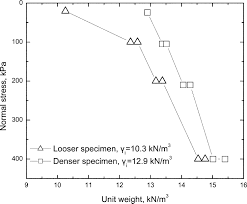The image above represents unit weight.

To compute for unit weight, three essential parameters are needed and these parameters are Bulk density of soil (g), Density of water (ρwand Unit Weight of water (γw).

The formula for calculating unit weight:

Γ = g . γw / ρw

Where:

Γ = Unit Weight
g = Bulk Density of Soil
ρw = Density of Water
γw = Unit Weight of Water

Let’s solve an example;
Find the unit weight when the bulk density of soil is 7, the density of water is 16 and the unit weight of water is 9.

This implies that;

g = Bulk Density of Soil = 7
ρw = Density of Water = 16
γw = Unit Weight of Water = 9

Γ = g . γw / ρw
Γ = (7) . (9) / 16
Γ = 63 / 16
Γ = 3.93

Therefore, the unit weight is 3.93.

Calculating for the Bulk Density of Soil when the Unit Weight, the Density of Water and the Unit Weight of Water is Given.

g = Γ x ρw / γw

Where;

g = Bulk Density of Soil
Γ = Unit Weight
ρw = Density of Water
γw = Unit Weight of Water

Let’s solve an example;
Find the bulk density of soil when the unit weight is 42, the density of water is 14 and the unit weight of water is 7.

This implies that;

Γ = Unit Weight = 42
ρw = Density of Water = 14
γw = Unit Weight of Water = 7

g = Γ x ρw / γw
g = 42 x 14 / 7
g = 588 / 7
g = 84

Therefore, the bulk density of soil is 84.

## How to Calculate and Solve for Specific Gravity of Soil Particle | Soil Mechanics and Foundation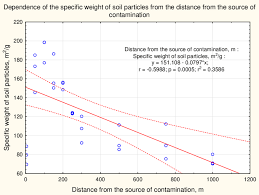The image above represents specific gravity of soil particle.

To compute for specific gravity of soil particle, two essential parameters are needed and these parameters are Density of water (ρwand Density of soil (ρs).

The formula for calculating specific gravity of soil particle:

Gs = ρs / ρw

Where:

Gs = Specific Gravity of Soil Particle
ρw = Density of Water
ρs = Density of Soil

Let’s solve an example;
Find the specific gravity of soil particle when the density of water is 22 and the the density of soil is 11.

This implies that;

ρw = Density of Water = 22
ρs = Density of Soil = 11

Gs = ρs / ρw
Gs = 11 / 22
Gs = 0.5

Therefore, the specific gravity of soil particle is 0.5.

Calculating the Density of Water when the Specific Gravity of Soil Particle and the Density of Soil is Given.

ρw = ρs / Gs

Where;

ρw = Density of Water
Gs = Specific Gravity of Soil Particle
ρs = Density of Soil

Let’s solve an example;
Given that the specific gravity of soil particle is 12 and the density of soil is 156. Find the density of water?

This implies that;

Gs = Specific Gravity of Soil Particle = 12
ρs = Density of Soil = 156

ρw = ρs / Gs
ρw = 156 / 12
ρw = 13

Therefore, the density of water is 13.

## How to Calculate and Solve for Water Content | Soil Mechanics and Foundation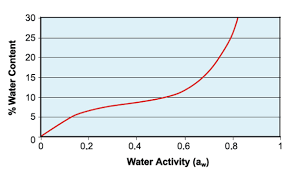The image above represents water content.

To compute for water content, two essential parameters are needed and these parameters are Mass of the water (Mwand Mass of the solid (Ms).

The formula for calculating water content:

w = Mw / Ms

Where:

w = Water Content
Mw = Mass of the Water
Ms = Mass of the Solid

Let’s solve an example;
Find the water content when the mass of the water is 28 and the mass of the solid is 7.

This implies that;

Mw = Mass of the Water = 28
Ms = Mass of the Solid = 7

w = Mw / Ms
w = 28 / 7
w = 4

Therefore, the water content is 4.

Calculating the Mass of the Water when the Water Content and the Mass of the Solid is Given.

Mw  = w x Ms

Where;

Mw = Mass of the Water
w = Water Content
Ms = Mass of the Solid

Let’s solve an example;
Find the mass of the water when the water content is 15 and mass of the solid is 4.

This implies that;

w = Water Content = 15
Ms = Mass of the Solid = 4

Mw  = w x Ms
Mw  = 15 x 4
Mw  = 60

Therefore, the mass of the water is 60.

## How to Calculate and Solve for Bulk Density | Soil Mechanics and Foundation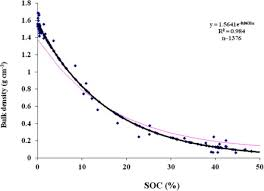The image above represents bulk density.

To compute for bulk density, two essential parameters are needed and these parameters are Mass of the soil (m) and Volume of the soil (V).

The formula for calculating bulk density:

sb = m / V

Where:

sb = Bulk Density
m = Mass of the Soil
V = Volume of the Soil

Let’s solve an example;
Find the bulk density when the mass of the soil is 24 and the volume of the soil is 6.

This implies that;

m = Mass of the Soil = 24
V = Volume of the Soil = 6

sb = m / V
sb = 24 / 6
sb = 4

Therefore, the bulk density is 4.

Calculating the Mass of the Soil when the Bulk Density and the Volume of the Soil is Given.

m = sb x V

Where;

m = Mass of the Soil
sb = Bulk Density
V = Volume of the Soil

Let’s solve an example;
Find the mass of the soil when the bulk density is 10 and the volume of the soil is 4.

This implies that;

sb = Bulk Density = 10
V = Volume of the Soil = 4

m = sb x V
m = 10 x 4
m = 40

Therefore, the mass of the soil  is 40.

## How to Calculate and Solve for Air Content of the Soil | Soil Mechanics and Foundation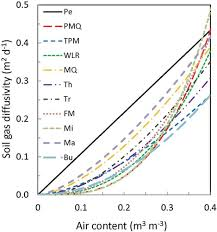The image above represents air content of the soil.

To compute for air content of the soil, two essential parameters are needed and these parameters are Volume of the Air (Vaand Volume of the soil (V).

The formula for calculating air content of the soil:

A = Va / V

Where:

A = Air Content of the Soil
Va = Volume of the Air
V = Volume of the Soil

Let’s solve an example;
Find the air content of the soil when the volume of the air is 20 and the volume of the soil is 24.

This implies that;

Va = Volume of the Air = 20
V = Volume of the Soil = 24

A = Va / V
A = 20 / 24
A = 0.83

Therefore, the air content of the soil is 0.83.

Calculating the Volume of the Air when the Air Content of the Soil and the Volume of the Soil is Given.

Va = A x V

Where;

Va = Volume of the Air
A = Air Content of the Soil
V = Volume of the Soil

Let’s solve an example;
Find the volume of the air when the air content of the soil is 12 and the volume of the soil is 3.

This implies that;

A = Air Content of the Soil = 12
V = Volume of the Soil = 3

Va = A x V
Va = 12 x 3
Va = 36

Therefore, the volume of the air is 36.

## How to Calculate and Solve for Porosity | Soil Mechanics and Foundation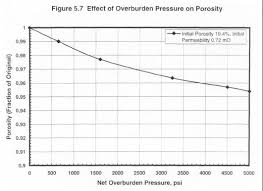The image above represents porosity.

To compute for porosity, two essential parameters are needed and these parameters are Volume of void (Vv) and Volume of the soil (V).

The formula for calculating porosity:

n = Vv / V

Where:

n = Porosity
Vv = Volume of Void
V = Volume of the Soil

Let’s solve an example;
Find the porosity when the volume of void is 12 and the volume of the soil is 14.

This implies that;

Vv = Volume of Void = 12
V = Volume of the Soil = 14

n = Vv / V
n = 12 / 14
n = 0.85

Therefore, the porosity is 0.85.

Calculating the Volume of Void when the Porosity and the Volume of the Soil is Given.

Vv = n x V

Where;

Vv = Volume of Void
n = Porosity
V = Volume of the Soil

Let’s solve an example;
Find the volume of void when the porosity is 21 and the volume of the soil is 7.

This implies that;

n = Porosity = 21
V = Volume of the Soil = 7

Vv = n x V
Vv = 21 x 7
Vv = 147

Therefore, the volume of the void is 147.

## How to Calculate and Solve for Void Ratio | Soil Mechanics and FoundationThe image above represents void ratio.

To compute for void ratio, two essential parameters are needed and these parameters are Volume of void (Vvand Volume of solid (Vs).

The formula for calculating void ratio:

e = Vv / Vs

Where:

e = Void Ratio
Vv = Volume of Void
Vs = Volume of Solid

Let’s solve an example;
Find the void ratio when the volume of void is 10 and the volume of solid is 20.

This implies that;

Vv = Volume of Void = 10
Vs = Volume of Solid = 20

e = Vv / Vs
e = 10 / 20
e = 0.5

Therefore, the void ratio is 0.5.

Calculating the Volume of Void when the Void Ratio and the Volume of Solid is Given.

Vv = e x Vs

Where;

Vv = Volume of Void
e = Void Ratio
Vs = Volume of Solid

Let’s solve an example;
Find the volume of void when the void ratio is 12 and the volume of solid is 5.

This implies that;

e = Void Ratio = 12
Vs = Volume of Solid = 5

Vv = e x Vs
Vv = 12 x 5
Vv = 60

Therefore, the volume of void is 60.